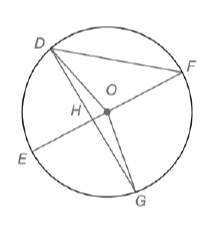Chapter 6.1, Problem 12E### Elementary Geometry for College St...

6th Edition
Daniel C. Alexander + 1 other
ISBN: 9781285195698

#### Solutions

Chapter
Section### Elementary Geometry for College St...

6th Edition
Daniel C. Alexander + 1 other
ISBN: 9781285195698
Textbook Problem
4 views

# Given: m ∠ D O E = 76 ∘ and m ∠ E O G = 82 ∘ in ⊙ O E F ¯  is a diameterFind: a) m D E ⌢ b) m D F ⌢ c) m ∠ F d) m ∠ D G E e) m ∠ E H G f) Whether m ∠ E H G = 1 2 ( m E G ⌢ + m D F ⌢ )

To determine

(a)

To calculate:

The mDE by using the provided figure.

Explanation

Given:

mDOE=76o and mEOG=82o in Q. EF¯ is a diameter.

Postulate used:

Central angle postulate:

In a circle, the degree of a central angle is equal to the degree measure of its intercepted arc.

Calculation:

Since, mDOE=76o

To determine

(b)

To calculate:

The mDF by using the provided figure.

To determine

(c)

To calculate: The F by using the provided figure.

To determine

(d)

To calculate:

The mDGE by using the provided figure.

To determine

(e)

To calculate:

The mEHG by using the provided figure.

To determine

(f)

To calculate:

The mEHG=12(mEG+mDF) by using the provided figure.

### Still sussing out bartleby?

Check out a sample textbook solution.

See a sample solution

#### The Solution to Your Study Problems

Bartleby provides explanations to thousands of textbook problems written by our experts, many with advanced degrees!

Get Started

#### Find more solutions based on key concepts